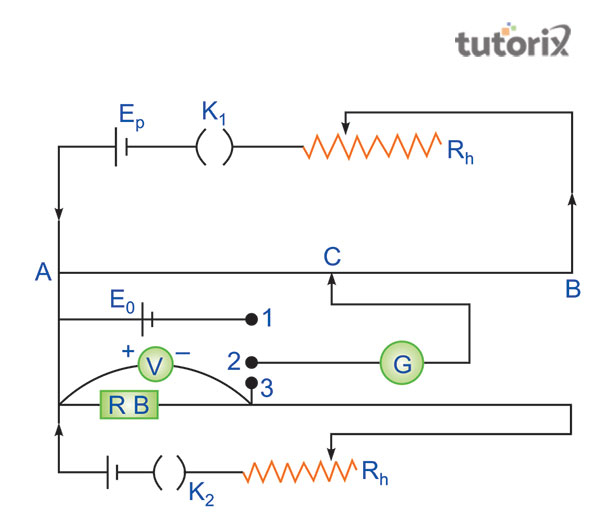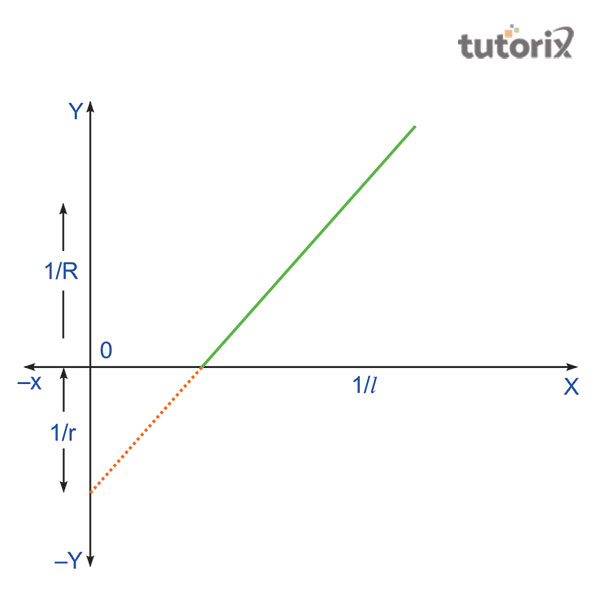# To determine the internal resistance of a given primary cell using a potentiometer experiment

Potentiometer is usually defined as a three-terminal resistor. This instrument has been generally applied for measuring the production of an adjustable voltage divider while rolling or sliding contact. The primary application of the Potentiometer is for the determination of the internal resistance of primary cells. In accordance with the theory of Thévenin, the power source can be acknowledged as a voltage source that can be ideal in series with impedance, usually termed as internal resistance.

## Aim

The main of this experiment is to determine the internal resistance of a given primary cell with the help of a potentiometer.

## Required apparatus

Among the required apparatus, the first requirement is a galvanometer. Voltameter, and potentiometer. The other requirements include a fractional resistance box, one battery, wires for the connection formation, and sandpaper (Batteryuniversity, 2022). Along with that, one must require two numbers keys that are of one way and one Leclanche cell. A jokey and one set square is required as well.

## Principle

In order to conduct the experiment, it needs to be conjectured that a resistance that is represented by the letter R is connected across the e.m.f of a cell. The internal resistance of this cell is represented by the letter r. Based on this, the current of the circuit that is I will be equivalent to the E = I(R+r) that can be represented as the ratio of E and R+t, which will be equivalent to I (Ncert, 2022). This mathematical representation of the theory leads to get the equation that is V = IR = E - Ir or r= (E/V -1)R.

In addition, it can be conjectured that the distance between the balance null points from the end A is l0 whereas l will be representative of the difference between the open and closed circuit of the potentiometer (Castaño et al. 2018). In such a case, the E will be proportional to l0 and the l will be proportional to V. Therefore, the formed equation of this experiment can be mathematically represented as r= (l0-l/l) R.

## Circuit diagramFigure 1: Diagram of the circuit

In order to start the experiment, one needs to connect different electrical components as shown in the above figure that represents the diagram of the circuit. The key K1 is required to be closed after checking the connections of the circuits (Barai et al. 2018). The protective high resistance that is represented by the letter P will be found in the position of the balance point after opening K3.

In order to get the final reading, one must be required to close the k3 for creating short-circuit the resistance P that will help to find the value of balance length l0.

In such cases, the R will be found to be equivalent to the 10 Ω. For measuring the new valance length l, one needs to close the k2. However, the k2 is required to be opened soon after this measurement (Pamies et al. 2018). Throughput the above observation, the readings must be kept in the ammeter. In this experiment, if the value of R will be reduced in equal steps of the 1 Ω, each of the values of R will obtain the balance length l. In order to find the value of l0, at the end of the experiment, the k2 needs to be opened along with repeating the second step of the experiment.

## Observations

Prior to the experiment, the value of l0 has been observed that must be found and represented with the unit of a centimetre. Therefore, it has been observed that the measurement of the e.m.f of the cell and the battery can also be determined through this experiment.

Sr. no Corrected reading of Ammeter Point of balance according to the E1 point of the circuit Point of balance when E2 in the circuit (E1/E2) = (l1/l2)

Table 1: Balance length

## Calculation and ResultFigure 2: Graph between 1/R and 1/l

In accordance with the above figure, the value of Eq can be represented as the ratio of R and 1 that will be equivalent to l0/r*(1/l)-1/r. this will be the equation of a straight line (Ncert, 2022). Taking the point l/1 on the x-axis and 1/R is positioned on the y-axis, the negative intercept on the y-axis will determine the value of 1/R hence, the value will be obtained is of r.

## Conclusion

The present tutorial has focused on the representation of the experiment for determining the internal resistance of a primary cell along with the help of a potentiometer. Based on this main, the tutorial has included numerous sections where the required materials and the precautions have been explained. Furthermore, the tutorial has included the explanation of the diagram and the calculation part explains the mathematical representation of the theory as well. The principle that has been used throughout the experiment is E = I(R+r).

## FAQs

Q1. What are some of the practical applications of potentiometer?

The practical applications of the potentiometer have included the machines like audio controllers, motion controllers, television and transducers.

Q2. What is the principle of the potentiometer?

The min principle, based on which the work process of the potentiometer is conducted is of a constant current. The principle of the potentiometer suggests that in a wire, the fall of potential is determined to be directly proportional to the length of the wire.

Q3. What is the e.m.f of a cell?

The abbreviation of electromotive force is represented as the e.m.f. This particular terminology of physics refers to the difference in potential across the cell’s potential at a time when the cell is considered an open circuit.

Q4. What should be the order of the magnitude of the e.m.f of an auxiliary battery?

It has been observed throughout the experience that the e.m.f of an auxiliary battery is greater in comparison to the individual cell.